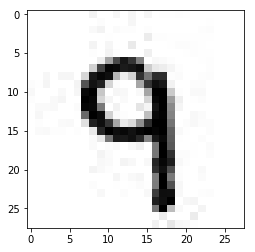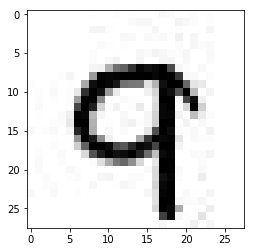Deep Learning Models -- A collection of various deep learning architectures, models, and tips for TensorFlow and PyTorch in Jupyter Notebooks.

In :
%load_ext watermark
%watermark -a 'Sebastian Raschka' -v -p tensorflow,numpy

Sebastian Raschka

CPython 3.6.1
IPython 6.0.0

tensorflow 1.2.0
numpy 1.13.0


# Chunking an Image Dataset for Minibatch Training using NumPy NPZ Archives¶

This notebook provides an example for how to organize a large dataset of images into chunks for quick access during minibatch learning. This approach uses NumPy .npz archive files and only requires having NumPy as a dependency so that this approach should be compatible with different Python-based machine learning and deep learning libraries and packages for further image (pre)processing and augmentation.

While this approach performs reasonably well (sufficiently well for my applications), you may also be interested in TensorFlow's "Reading Data" guide to work with TfRecords and file queues.

## 0. The Dataset¶

Let's pretend we have a directory of images containing two subdirectories with images for training, validation, and testing. The following function will create such a dataset of images in JPEG format locally for demonstration purposes.

In :
# Note that executing the following code
# and save all the 60,000 images as separate JPEG
# files. This might take a few minutes depending

import numpy as np

import sys
sys.path.insert(0, '..')
from helper import mnist_export_to_jpg

np.random.seed(123)
mnist_export_to_jpg(path='./')

Extracting ./train-images-idx3-ubyte.gz
Extracting ./train-labels-idx1-ubyte.gz
Extracting ./t10k-images-idx3-ubyte.gz
Extracting ./t10k-labels-idx1-ubyte.gz

In :
import os

for i in ('train', 'valid', 'test'):
print('mnist_%s subdirectories' % i, os.listdir('mnist_%s' % i))

mnist_train subdirectories ['0', '1', '2', '3', '4', '5', '6', '7', '8', '9']
mnist_valid subdirectories ['0', '1', '2', '3', '4', '5', '6', '7', '8', '9']
mnist_test subdirectories ['0', '1', '2', '3', '4', '5', '6', '7', '8', '9']


Note that the names of the subdirectories correspond directly to the class label of the images that are stored under it.

To make sure that the images look okay, the snippet below plots an example image from the subdirectory mnist_train/9/:

In :
%matplotlib inline
import matplotlib.image as mpimg
import matplotlib.pyplot as plt
import os

some_img = os.path.join('./mnist_train/9/', os.listdir('./mnist_train/9/'))

print(img.shape)
plt.imshow(img, cmap='binary');

(28, 28)Note: The JPEG format introduces a few artifacts that we can see in the image above. In this case, we use JPEG instead of PNG. Here, JPEG is used for demonstration purposes since that's still format many image datasets are stored in.

## 1. Chunking Images into NumPy NPZ Archive Files¶

The following wrapper function creates .npz archive files training, testing, and validation. It will group images together into integer arrays that are then saved as .npz archive files. The number of rows (images) in each .npz archive will be equal to the archive_size argument.

In :
import numpy as np
import glob

def images_to_pickles(data_stempath='./mnist_', which_set='train',
archive_size=5000, width=28, height=28, channels=1,
shuffle=False, seed=None):

if not os.path.exists('%snpz' % data_stempath):
os.mkdir('%snpz' % data_stempath)

img_paths = [p for p in glob.iglob('%s%s/**/*.jpg' %
(data_stempath, which_set), recursive=True)]
if shuffle:
rgen = np.random.RandomState(seed)
paths = rgen.shuffle(img_paths)

idx, file_idx = 0, 1
data = np.zeros((archive_size, height, width, channels), dtype=np.uint8)
labels = np.zeros(archive_size, dtype=np.uint8)
for path in img_paths:
if idx >= archive_size - 1:
idx = 0
savepath = os.path.join('%snpz' % data_stempath, '%s_%d.npz' %
(which_set, file_idx))
file_idx += 1
np.savez(savepath, data=data, labels=labels)

label = int(os.path.basename(os.path.dirname(path)))

if len(image.shape) == 2:
data[idx] = image[:, :, np.newaxis]
labels[idx] = label
idx += 1

In :
images_to_pickles(which_set='train', shuffle=True, seed=1)
images_to_pickles(which_set='valid', shuffle=True, seed=1)
images_to_pickles(which_set='test', shuffle=True, seed=1)


The .npz files we created are stored under a new directory, mnist_npz:

In :
os.listdir('mnist_npz')

Out:
['test_1.npz',
'test_2.npz',
'train_1.npz',
'train_2.npz',
'train_3.npz',
'train_4.npz',
'train_5.npz',
'train_6.npz',
'train_7.npz',
'train_8.npz',
'train_9.npz',
'valid_1.npz']

To check that the archiving worked correctly, we will now load one of those .npz archives. Note that we can now access each archive just like a python dictionary. Here the 'data' key contains the image data and the 'labels' key stores an array containing the corresponding class labels:

In :
data = np.load('mnist_npz/test_1.npz')
print(data['data'].shape)
print(data['labels'].shape)

(5000, 28, 28, 1)
(5000,)

In :
plt.imshow(data['data'][:, :, -1], cmap='binary');
print('Class label:', data['labels'])

Class label: 9The following cell implements a class for iterating over the MNIST images, based on the .npz archives, conveniently. Via the normalize parameter we additionally scale the image pixels to [0, 1] range, which typically helps with gradient-based optimization in practice.

The key functions (here: generators) are

These let us iterate over small chunks (determined via minibatch_size). Each of these functions will load the images from a particular .npz archive into memory (here: 5000 images) and yield minibatches of smaller or equal size (for example, 50 images at a time). Via the two shuffle parameters, we can further control if the images within each .npz archive should be shuffled, and if the order the .npz files are loaded should shuffled after each epoch. By setting onehot=True, the labels are converted into a onehot representation for convenience.

In :
class BatchLoader():
def __init__(self, minibatches_path,
normalize=True):

self.normalize = normalize

self.train_batchpaths = [os.path.join(minibatches_path, f)
for f in os.listdir(minibatches_path)
if 'train' in f]
self.valid_batchpaths = [os.path.join(minibatches_path, f)
for f in os.listdir(minibatches_path)
if 'valid' in f]
self.test_batchpaths = [os.path.join(minibatches_path, f)
for f in os.listdir(minibatches_path)
if 'train' in f]

self.num_train = 45000
self.num_valid = 5000
self.num_test = 10000
self.n_classes = 10

shuffle_within=False, shuffle_paths=False,
seed=None):
batch_size=batch_size,
onehot=onehot,
shuffle_within=shuffle_within,
shuffle_paths=shuffle_paths,
seed=seed):
yield batch_x, batch_y

shuffle_within=False, shuffle_paths=False,
seed=None):
batch_size=batch_size,
onehot=onehot,
shuffle_within=shuffle_within,
shuffle_paths=shuffle_paths,
seed=seed):
yield batch_x, batch_y

shuffle_within=False, shuffle_paths=False,
seed=None):
batch_size=batch_size,
onehot=onehot,
shuffle_within=shuffle_within,
shuffle_paths=shuffle_paths,
seed=seed):
yield batch_x, batch_y

shuffle_within=True, shuffle_paths=True, seed=None):

if which == 'train':
paths = self.train_batchpaths
elif which == 'valid':
paths = self.valid_batchpaths
elif which == 'test':
paths = self.test_batchpaths
else:
raise ValueError('which must be "train" or "test". Got %s.' %
which)

rgen = np.random.RandomState(seed)
if shuffle_paths:
paths = rgen.shuffle(paths)

for batch in paths:

if onehot:
labels = (np.arange(self.n_classes) ==
dct['labels'][:, None]).astype(np.uint8)
else:
labels = dct['labels']

if self.normalize:
# normalize to [0, 1] range
data = dct['data'].astype(np.float32) / 255.
else:
data = dct['data']

arrays = [data, labels]
del dct
indices = np.arange(arrays.shape)

if shuffle_within:
rgen.shuffle(indices)

for start_idx in range(0, indices.shape - batch_size + 1,
batch_size):
index_slice = indices[start_idx:start_idx + batch_size]
yield (ary[index_slice] for ary in arrays)


The following for loop will iterate over the 45,000 training examples in our MNIST training set, yielding 50 images and labels at a time (note that we previously set aside 5000 training example as our validation datast).

In :
batch_loader = BatchLoader(minibatches_path='./mnist_npz/',
normalize=True)

print(batch_x.shape)
print(batch_y.shape)
break

(50, 28, 28, 1)
(50, 10)

In :
cnt = 0
cnt += batch_x.shape

print('One training epoch contains %d images' % cnt)

One training epoch contains 45000 images

In :
def one_epoch():
pass

% timeit one_epoch()

249 ms ± 14 ms per loop (mean ± std. dev. of 7 runs, 1 loop each)


As we can see from the benchmark above, an iteration over one training epoch (45k images) is relatively fast.

Similarly, we could iterate over validation and test data via

## 3. Training a Model using TensorFlow's feed_dict¶

The following code demonstrate how we can feed our minibatches into a TensorFlow graph using a TensorFlow session's feed_dict.

### Multilayer Perceptron Graph¶

In :
import tensorflow as tf

##########################
### SETTINGS
##########################

# Hyperparameters
learning_rate = 0.1
training_epochs = 15
batch_size = 100

# Architecture
n_hidden_1 = 128
n_hidden_2 = 256
height, width = 28, 28
n_classes = 10

##########################
### GRAPH DEFINITION
##########################

g = tf.Graph()
with g.as_default():

tf.set_random_seed(123)

# Input data
tf_x = tf.placeholder(tf.float32, [None, height, width, 1], name='features')
tf_x_flat = tf.reshape(tf_x, shape=[-1, height*width])
tf_y = tf.placeholder(tf.int32, [None, n_classes], name='targets')

# Model parameters
weights = {
'h1': tf.Variable(tf.truncated_normal([width*height, n_hidden_1], stddev=0.1)),
'h2': tf.Variable(tf.truncated_normal([n_hidden_1, n_hidden_2], stddev=0.1)),
'out': tf.Variable(tf.truncated_normal([n_hidden_2, n_classes], stddev=0.1))
}
biases = {
'b1': tf.Variable(tf.zeros([n_hidden_1])),
'b2': tf.Variable(tf.zeros([n_hidden_2])),
'out': tf.Variable(tf.zeros([n_classes]))
}

# Multilayer perceptron
layer_1 = tf.nn.relu(layer_1)
layer_2 = tf.nn.relu(layer_2)
out_layer = tf.matmul(layer_2, weights['out']) + biases['out']

# Loss and optimizer
loss = tf.nn.softmax_cross_entropy_with_logits(logits=out_layer, labels=tf_y)
cost = tf.reduce_mean(loss, name='cost')
train = optimizer.minimize(cost, name='train')

# Prediction
correct_prediction = tf.equal(tf.argmax(tf_y, 1), tf.argmax(out_layer, 1))
accuracy = tf.reduce_mean(tf.cast(correct_prediction, tf.float32), name='accuracy')


### Training the Neural Network with Minibatches¶

In :
##########################
### TRAINING & EVALUATION
##########################

normalize=True)

# by unpacking the generator
onehot=True)
valid_x, valid_y = valid_data, valid_data
del valid_data

with tf.Session(graph=g) as sess:
sess.run(tf.global_variables_initializer())

for epoch in range(training_epochs):
avg_cost = 0.

n_batches = 0
onehot=True,
seed=epoch):
n_batches += 1
_, c = sess.run(['train', 'cost:0'], feed_dict={'features:0': batch_x,
'targets:0': batch_y.astype(np.int)})
avg_cost += c

train_acc = sess.run('accuracy:0', feed_dict={'features:0': batch_x,
'targets:0': batch_y})

valid_acc = sess.run('accuracy:0', feed_dict={'features:0': valid_x,
'targets:0': valid_y})

print("Epoch: %03d | AvgCost: %.3f" % (epoch + 1, avg_cost / n_batches), end="")
print(" | MbTrain/Valid ACC: %.3f/%.3f" % (train_acc, valid_acc))

# imagine test set is too large to fit into memory:
test_acc, cnt = 0., 0
onehot=True):
cnt += 1
acc = sess.run(accuracy, feed_dict={'features:0': test_x,
'targets:0': test_y})
test_acc += acc
print('Test ACC: %.3f' % (test_acc / cnt))

Epoch: 001 | AvgCost: 0.467 | MbTrain/Valid ACC: 0.960/0.928
Epoch: 002 | AvgCost: 0.222 | MbTrain/Valid ACC: 0.970/0.944
Epoch: 003 | AvgCost: 0.166 | MbTrain/Valid ACC: 0.980/0.951
Epoch: 004 | AvgCost: 0.134 | MbTrain/Valid ACC: 0.990/0.959
Epoch: 005 | AvgCost: 0.112 | MbTrain/Valid ACC: 0.990/0.962
Epoch: 006 | AvgCost: 0.096 | MbTrain/Valid ACC: 0.990/0.964
Epoch: 007 | AvgCost: 0.083 | MbTrain/Valid ACC: 0.990/0.967
Epoch: 008 | AvgCost: 0.073 | MbTrain/Valid ACC: 0.990/0.966
Epoch: 009 | AvgCost: 0.064 | MbTrain/Valid ACC: 0.990/0.967
Epoch: 010 | AvgCost: 0.057 | MbTrain/Valid ACC: 0.990/0.970
Epoch: 011 | AvgCost: 0.050 | MbTrain/Valid ACC: 0.990/0.970
Epoch: 012 | AvgCost: 0.044 | MbTrain/Valid ACC: 0.990/0.970
Epoch: 013 | AvgCost: 0.039 | MbTrain/Valid ACC: 0.990/0.971
Epoch: 014 | AvgCost: 0.035 | MbTrain/Valid ACC: 0.990/0.971
Epoch: 015 | AvgCost: 0.031 | MbTrain/Valid ACC: 0.990/0.971
Test ACC: 0.992